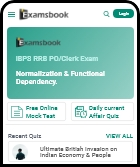12405 1

• 1
75 litres
• 2
100 litres
• 3
150 litres
• 4
120 litres
• Workspace

## Answer : 4. "120 litres" Explanation :Answer: D) 120 litres Explanation: wine(left)wine(added) = 343169   It means  wine(left)wine(initial amount) = 343512    (since 343 + 169 = 512)    Thus,  343x = 512x1 - 15k3    343512 = 783 = 1 - 15k3    1-15k=78=1-18    Thus the initial amount of wine was 120 liters.

### Are you sure